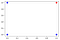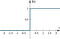# Implementing the Perceptron Learning Algorithm to Solve AND Gate in Python

If you searched for Neural Networks, Deep Learning, Machine Learning, or anything that has to do with Artificial Intelligence, you’ve probably heard about the Perceptron. The1958’s famous algorithm created by Frank Rosenblatt is the landing mark in Neural Networks and consequently Deep Learning, and understanding the way it works (i.e., the math behind it) is essential to go a step further to more complex IA models, specifically in Neural Networks.

# AND gate as a classification problem

We can say that all a perceptron does is apply some transformations (mathematical functions) in a set of inputs to represent them in a significant way in space (actually, that’s all that any machine learning algorithm does, but keep that between us). Besides the transforms, we need one more step to make things work: the learning step. The learning step checks if our transformation is working fine. for that, we need the answer to our problem to compare if our actual output is correct (or close to the correct answer). To illustrate, look at the truth table of the AND gate.

we have a set of inputs ( [0,0], [0,1], [1,0], [1,1]) and their respective outputs ([0, 0, 0, 1]) that we can consider their labels in a machine learning problem. We can represent the AND gate in geometric way, using the inputs as coordinates and the outputs as characteristics of these points (like colors):AND gate represented in a bi-dimensional space. the outputs are associated with colors

For each input, we have a point in space with coordinates (x, y), and the colors of the points represent the output of AND gate (blue if output = 0, red if input = 1). So that gives us a classification problem (we have two classes of points, blue and red (or AND output 0 and 1), and we want to separate the space accordingly with these classes, like that:

# Perceptron Algorithm

We want an algorithm that finds for us the adequate line, as already said. If you look closely at the perceptron structure image, you can identify the steps to search for this line:

2. apply a linear transformation (with the weights w_1, w_2, theta)
3. produce an output based on the previously transformation

The transform applies is a linear combination in the real-valued vector of inputs, that is:

we gonna get a scalar output for this transform, and then, we pass it a function that is called the activation function. In particular, Perceptron uses a unit step function (also known as Heaviside function), defined by:

In other words, it gives to us a binary outcome. if the sum of our linear combination is greater than 0, we take 1 as an answer, else, we get zero. (exactly the same classes that we have in the AND gate).

Heaviside’s plot look’s like:graph representation of unit step function (here H -> f, x -> t, from the above equation)

it acts as a filter to us. So, with perceptron, we have the mechanism to receive the inputs from the AND gate and return a label! after doing the linear transform and applying the unit step function, we just need to compare with the actual answers from the AND gate and check if the transform we applied is correctly giving us the corresponding outputs, or, in other words, correctly separating the space!

# Actual Implementation

Let’s build the algorithm to solve this. Our separator line defines two areas in the space that differentiate blue dots from red dots. Note that our linear transformation in the inputs of the perceptron actually defines a plane:

So when we implement perceptron with 2-coordinate inputs we actually searching for a plane in 3d space like this:

But, we know that the AND answers lie’s in the x-y plane, so we simply project this plane in x-y plane, and obtain the line we desire:

Our problem with the AND port is to find the correct w1, w2, and theta values to build the line separating the blue points from the red point in the plane. The algorithm will start with random weights values (w_1, w_2, theta) and at each cycle of applying transformations in the inputs (also called epochs), it compares with the correct known answers. if we are getting the wrong answers, the algorithm changes the values of the weights and repeats the process.

We start with random values of the weights w1, w2, and theta:

`w = np.random.rand(1,3) * 10w_1 = np.round(w, 1)w_2 = np.round(w, 1)theta = np.round(w, 1)`

we need our inputs and known answers:

`x = [ [0,0], [0,1], [1,0], [1,1]]x_array = np.asarray(x)# expected outputs (AND port is the product of each entryout = x_array[:, 1] * x_array[:, 0]`

then we also need our Heaviside or step function:

`#step function def step (net):    if net >= 0:        return 1    else:        return 0`

We can plot our initialized line to see how it looks like:

So, it's clearly poorly separating our points. What the Perceptron algorithm does is adjust the weights until it finds a better line. each step trought all the inputs is called an ‘epoch’. we can check if our output is wrong by subtracting the correct answer from the output. We do this for the initial error:

`#the error vector error = np.array([0,0,0,0])for i in range(len(x)):    f_net = step(np.dot(np.asarray([w_1, w_2]) , x[i])  + theta)    error[i] = out[i] - f_netE = np.sum(error)`

The algorithm will run untill converges to the right line or we run a max number of iterations

`max_it = 1000t = 1learning_rate=0.1vals = [[w_1, w_2, theta]]while t < max_it & E != 0:    for i in range(len(x)):        f_net = step(np.dot(np.asarray([w_1, w_2]) , x[i])  + theta)        error[i] = out[i] - f_net        w_1 = w_1 + learning_rate * error[i] * x[i]        w_2 = w_2 + learning_rate * error[i] * x[i]        theta = theta + learning_rate*error[i]        vals.append([w_1, w_2, theta])    E = np.sum(error)   # print('sum of errors', E)    t = t+1`

The learning rate parameter controls the ‘size’ of correction of each parameter, a small value can make the algorithm too slow, and a big one can get in the way of converging. we use the ‘vals’ vector to store each new value of weights, so we can generate an animation of what's going on in the algorithm. Lets look at the code in more detail

`for i in range(len(x)):        f_net = step(np.dot(np.asarray([w_1, w_2]) , x[i])  + theta)        error[i] = out[i] - f_net`

Or four loop is responsible for one ‘epoch’ of the algorithm, in particular, we have four different input vectors ( (0,0), (0,1), (1,0), (1,1) ), so our loop runs 4 times. the ‘f_net’ parameter is the whole transformation applied in the inputs, including the step function filtering. it will always be 0 or 1, so when we calculate the error, we only get -1 or 0.

`w_1 = w_1 + learning_rate * error[i] * x[i]        w_2 = w_2 + learning_rate * error[i] * x[i]        theta = theta + learning_rate*error[i]`

this is the correction step. notice that if error = 0 we do nothing because the parameter is correct. theta always get corrected because it doesn't depend on the input value (is the linear coefficient of our line).

`vals.append([w_1, w_2, theta])    E = np.sum(error)`

to finish the cycle we save the weight values and sum each error, and until this sum doesn't go to zero, we repeat the process. we can see what's happening in the animation below:

For now that's it my friends, hope it gives a feeling of how a basic learning algorithm works. any questions, concerns, and critics are very welcome, see ya!

## Analytics Vidhya

### By Analytics Vidhya

Latest news from Analytics Vidhya on our Hackathons and some of our best articles! Take a look

Written by

Written by

## Neto Figueira

#### Physicist, Data Scientist